# java面向对象（一）

### 目录

• 1.Java面向对象学习的三条主线
• 2.面向对象与面向过程（人把大象装进冰箱）
• 2.1面向过程
• 2.2面向对象
• 3.面向对象的两个要素：类和对象
• 3.1类
• 3.1.1属性
• 属性 vs 局部变量
• 3.1.2方法
• 3.2对象
• 对象数组
• 匿名对象
• 3.3内存解析
• 4.方法
• 4.2可变个数的形参
• 4.3值传递机制
• 4.3.1关于变量的赋值
• 4.3.2方法形参的值传递机制
• 4.4递归方法
• 5.封装性（封装和隐藏）
• 5.1问题的引入
• 5.2封装性的体现
• 5.3四种权限修饰符
• 6.构造器（构造方法）
• 6.1构造器的作用：
• 6.2总结属性赋值的先后顺序
• 7.JavaBean
• 8.UML类图
• 9.this关键字
• 10.package关键字
• 11.import关键字
• 12.MVC设计模式

# 1.Java面向对象学习的三条主线

1. Java类及类的成员：属性、方法、构造器：代码块、内部类
2. 面向对象三大特征：封装、继承、多态
3. 其他关键字：this、super、static、final、abstract、interface、package、import

# 2.面向对象与面向过程（人把大象装进冰箱）

### 2.1面向过程

``````1.把冰箱门打开
2.抬起大象，塞进冰箱
3.把冰箱门关闭
``````

### 2.2面向对象

``````人{
打开（冰箱）{
冰箱.开开（）；
}
抬起（大象）{
大象.进入（冰箱）；
}
关闭（冰箱）{
冰箱.闭合（）；
}
}

开开（）{}
闭合（）{}
}

进入（冰箱）{}
}
``````

# 3.面向对象的两个要素：类和对象

``````//1.创建类，设计类的成员
class Person{
//属性
String name;
int age;
boolean isMale;
//方法
public void eat(){
System.out.println("人可以吃饭");
}
}
//测试类
public class PersonTest{
public static void main(String[] args){
//2.创建Person类的对象
Person p1 = new Person();
Scaner scanner = new Scanner(System.in);
//3.调用属性:对象.属性：
p1.name = "Tom";
//4.调用方法:对象.方法；
p1.eat();
}
}
``````

## 3.1类

### 3.1.1属性

#### 属性 vs 局部变量

1.在类中声明的位置不同

2.关于权限修饰符(private,public,缺省,protected)的不同

3.默认初始化值的情况

4.在内存中加载的位置

}

## 3.2对象

“万事万物皆对象”
1.在java语言范畴中，我们都将功能、结构等封装到类中，通过类的实例化，来调用具体的功能结构。
2.涉及到java语言与前端html、后端数据库交互时，前后端的结构在java层面交互时，都体现类、对象。

### 对象数组

1.生成随机数：Math.random()，返回值类型为double
2.四舍五入取整：Math.round(double d)，返回值类型long

``````public class demo1 {
public static void main(String[] args) {
//声明Student类型的数组
Student[] st=new Student;
for(int i=0;i<20;i++) {
//给数组元素赋值
st[i] = new Student();
//给Student对象的属性赋值
st[i].num = i + 1;
//Math.random()范围[0.0,1.0),返回值为double
st[i].state = (int) (Math.random() * 6 + 1);
//System.out.println(st[i].state);
st[i].score = (int) (Math.random() * 100);
}
demo1 d=new demo1();
d.search(st,3);
d.sort(st);
}
//查找指定年级的学生信息
public void search(Student st[],int sta){
for(int i=0;i<st.length;i++){
if (st[i].state == sta) System.out.println(st[i].Info());
}
}
//对学生成绩使用冒泡排序按从低到高排序
public void sort(Student st[]){
for(int i=0;i<st.length-1;i++){
for(int j=0;j<st.length-1-i;j++){
if(st[j].score>st[j+1].score){
Student temp=st[j];
st[j]=st[j+1];
st[j+1]=temp;
}
}
}
print(st);
}
//输出学生信息
public void print(Student st[]){
for(int i=0;i<st.length;i++){
System.out.println(st[i].Info());
}
}
}
class Student{
int num;//学号
int state;//年级
int score;//成绩
String Info(){
return ("学号："+num+" 年级："+state+" 成绩："+score);
}
}
``````

### 匿名对象

``````new Student().num=10;
new Student().Info();
``````

## 3.3内存解析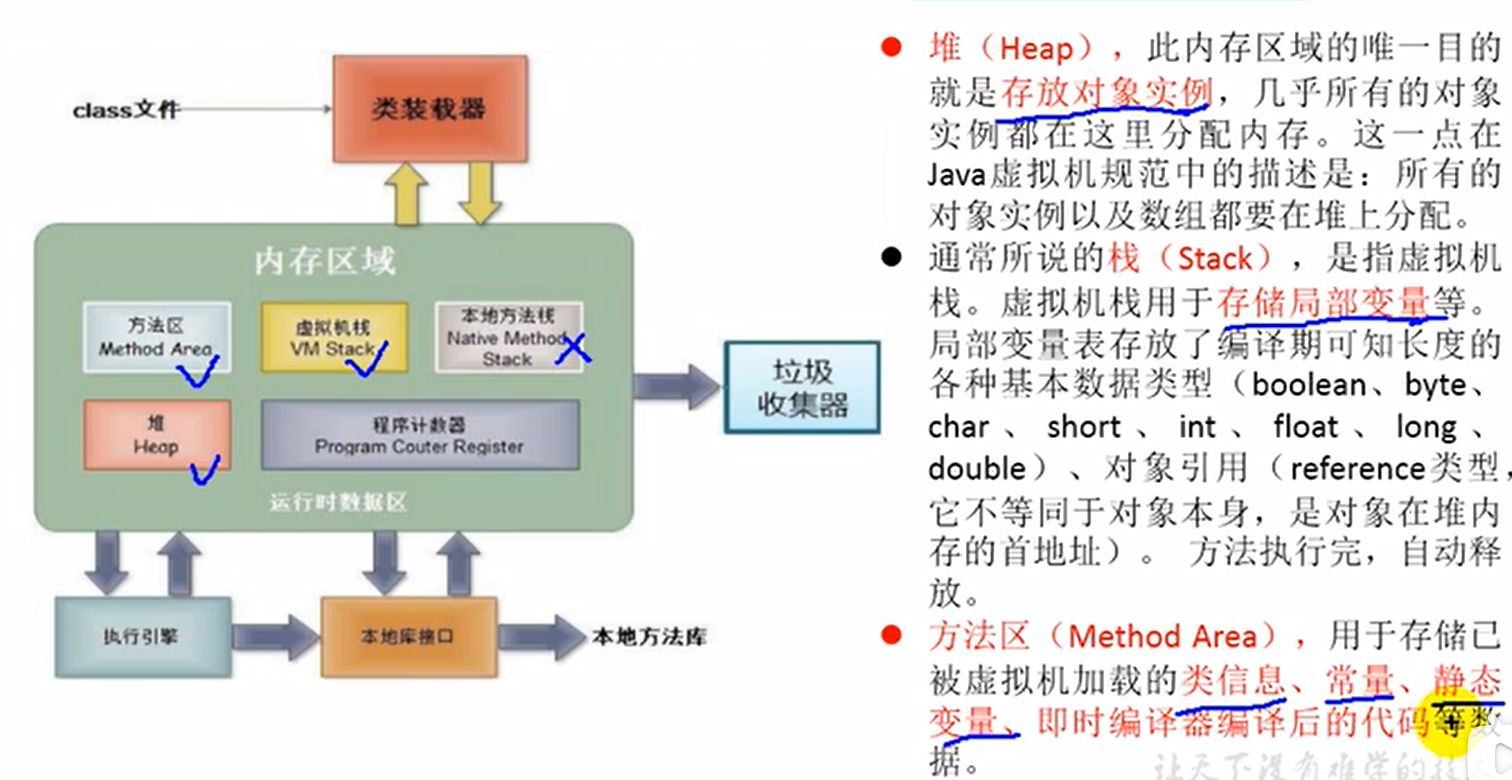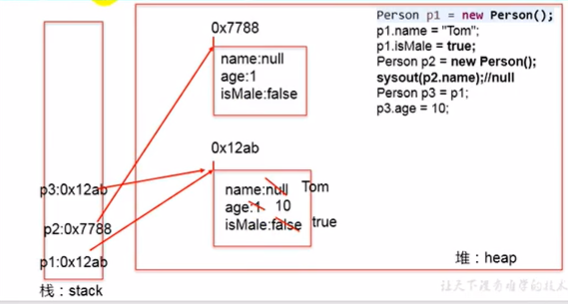# 4.方法

System.out.println()就是一个典型的重载方法。

## 4.2可变个数的形参

``````public class demo2 {
public static void main(String[] args) {
demo2 d=new demo2();
d.show(1);
d.show("h");
d.show("h1","h2");
d.show();
d.show(new String[]{"aa","bb","cc"});
}
public void show(int i){}
public void show(String s){}
//可变个数的形参举例
public void show(String ...str){
for(int i=0;i<str.length;i++){
System.out.println(str[i]);
}
}
public void show(String[] str){}//与上一个方法不能同时存在，因为它们是相同的方法，不构成重载
}
``````

``````public void show(int i,String...str){}
``````

## 4.3值传递机制

### 4.3.1关于变量的赋值

1.变量是基本数据类型

``````int m=1;
int n=m;
n=2;
//此时m为1，n为2
``````

2.变量是引用数据类型

``````//Order为一个类，有属性num
Order o1=new Order();
o1.num=1001;
Order o2=o1;
o2=1002;
//此时o1和o2都是1002
``````

### 4.3.2方法形参的值传递机制

1.形参是基本数据类型，此时实参赋给形参的是实参真实存储的数据值。

``````public class demo3 {
public static void main(String[] args) {
int m=10;
int n=20;
demo3 d=new demo3();
d.swap(m,n);
System.out.println("m="+m+" n="+n);//输出m=10 n=20
}
public void swap(int m,int n){
int swap=m;
m=n;
n=swap;
}
}
``````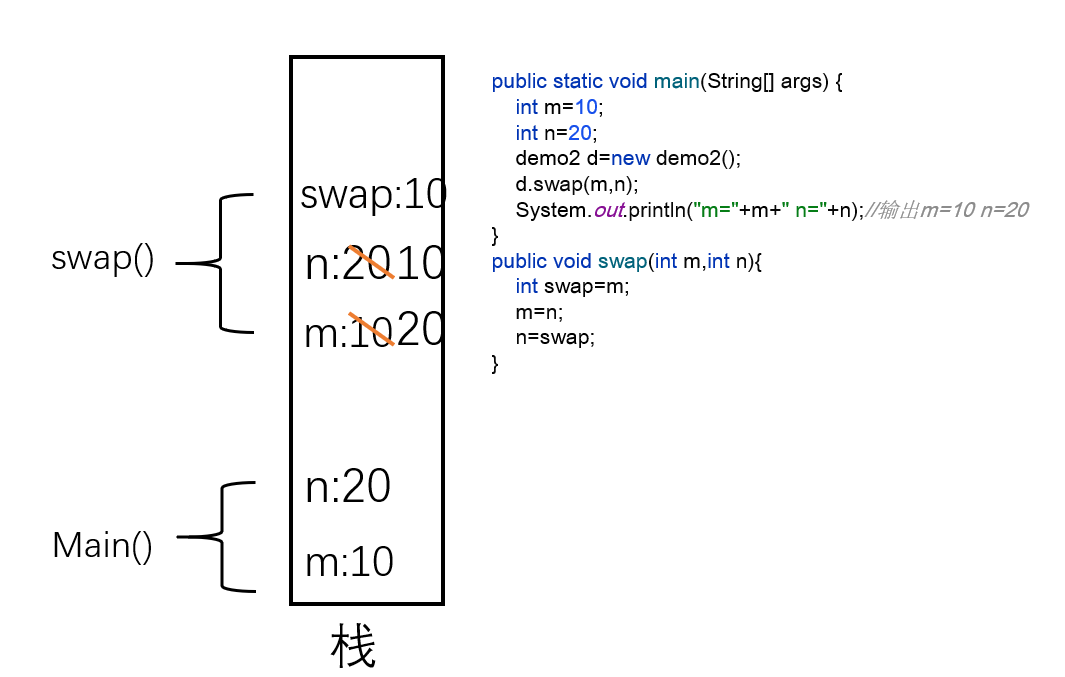swap()调用结束后，栈中swap()的局部变量释放（出栈），在main()中输出的m,n仍是栈中的m,n的值。
2.参数是引用数据类型，此时实参赋给形参的是实参存储数据的地址值。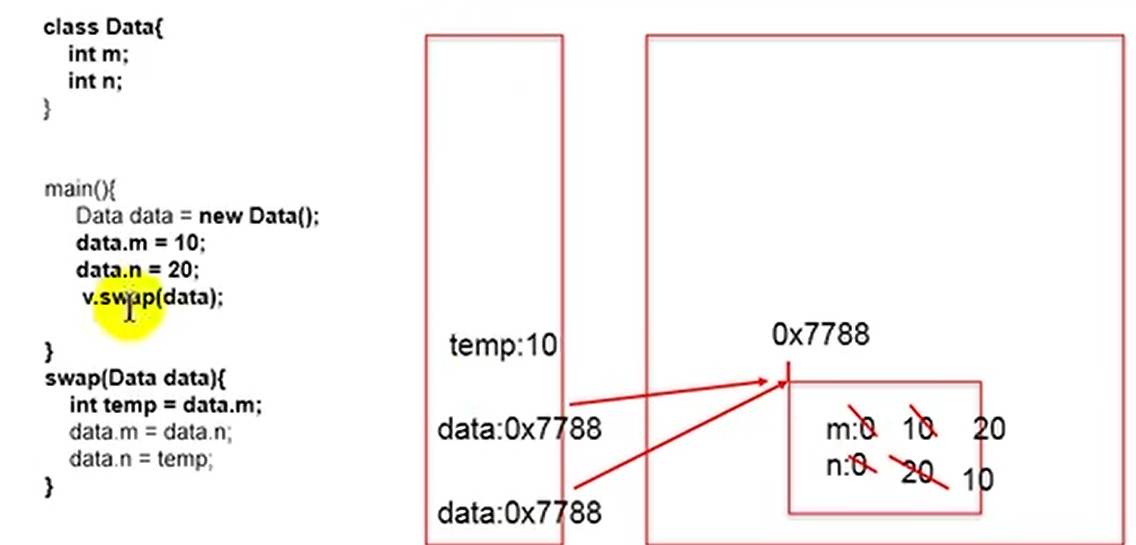swap()调用结束后，栈顶temp和data出栈，栈中只剩下main()中的data，此时data指向堆中已经修改后的对象。因此成功交换m,n

## 4.4递归方法

``````public class demo4 {
public static void main(String[] args) {
demo4 d=new demo4();
System.out.println(d.digui(10));//输出10497
}
public int digui(int n){
if(n==0)return 1;
else if(n==1)return 4;
else{
return 2*digui(n-1)+digui(n-2);
}
}
}
``````

``````public class demo5 {
public static void main(String[] args) {
demo5 d=new demo5();
System.out.println(d.digui(10));
}
public int digui(int n){
if(n==1||n==2)return 1;
else{
return digui(n-1)+digui(n-2);
}
}
}
``````

# 5.封装性（封装和隐藏）

## 5.2封装性的体现

1.将类的属性(xxx)私有化(private)，同时，提供公共的(public)方法来获取(getXxx)和设置(setXxx)此属性的值。

``````public class AnimalTest {
public static void main(String[] args) {
Animal an=new Animal();
an.name="小狗";
an.age=1;
an.setLegs(4);
an.getLegs();
an.print();
}
}
class Animal{
String name;
int age;
private int legs;//动物腿的条数
//限制对动物腿数的赋值
public void setLegs(int l){
if(l>=0&&l%2==0) legs=l;
else legs=0;
}
//获取动物腿的个数
public int getLegs(){
return legs;
}
public void print(){
System.out.println("name:"+name+" age:"+age+" legs:"+legs);
}
}
``````

2.将类的方法私有化，仅供类内使用
3.单例模式
……

## 5.3四种权限修饰符

privateyes

protectedyesyesyes
publicyesyesyesyes

# 6.构造器（构造方法）

## 6.1构造器的作用：

1.创建对象

``````public class PersonTest {
public static void main(String[] args) {
Person p=new Person();//Person()就是构造器，是系统提供的默认的空参构造器
p.setAge(12);
}
}
public class Person {
private int age;
public void setAge(int a){
age=a;
}
public int getAge(){
return age;
}
}
``````

2.初始化对象的信息

``````public class PersonTest {
public static void main(String[] args) {
Person p=new Person("Tom");
}
}
public class Person {
private int age;
String name;
//构造器
public Person(){
System.out.println("Person()");
}
public Person(String n){
name=n;
}
}
``````

## 6.2总结属性赋值的先后顺序

1.默认初始化值
2.显式初始化
3.构造器赋值
4.通过“对象.方法”或“对象.属性”的方式赋值

# 7.JavaBean

JavaBean是一种java语言写成的可重用组件

JavaBean是符合以下标准的java类：
1.类是公共的
2.有一个无参的公共构造器
3.有属性，且有对应的get、set方法

……待更

# 8.UML类图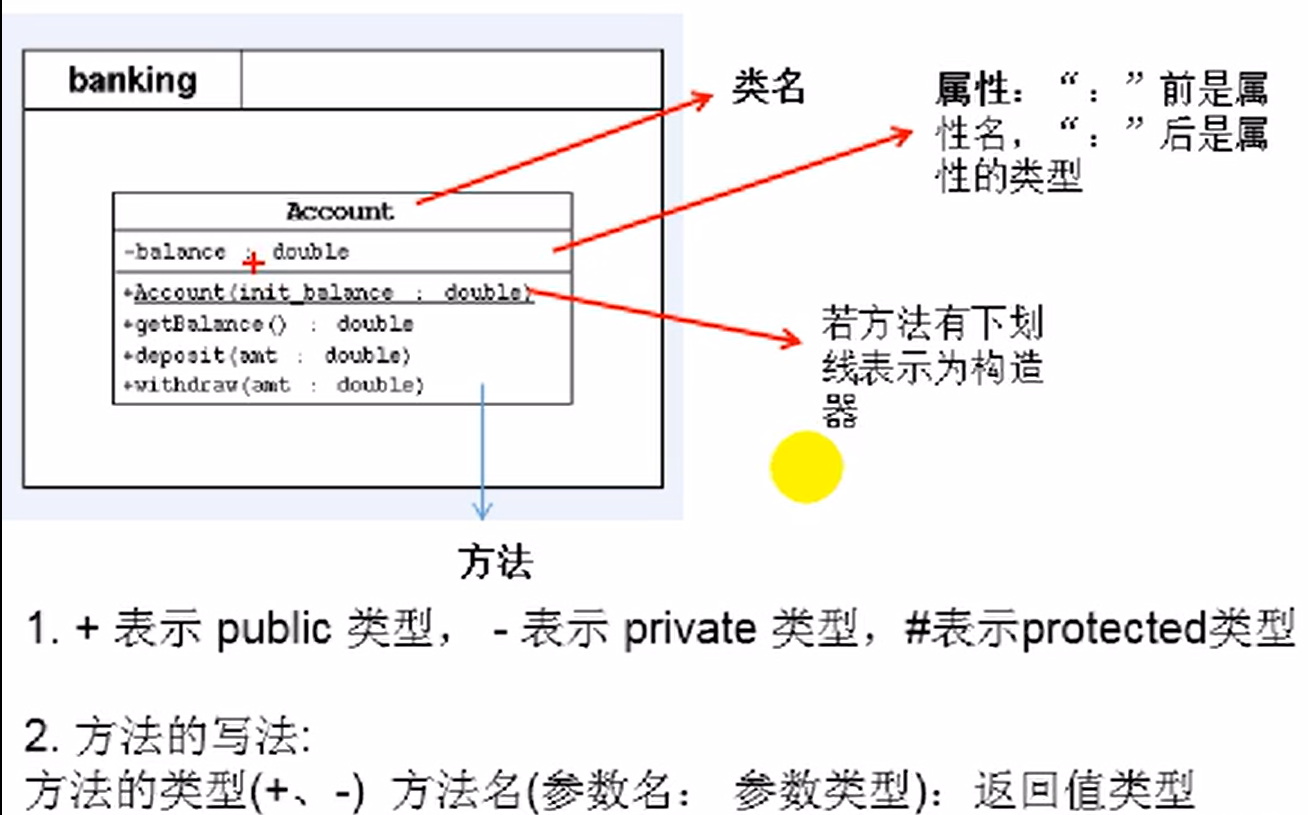# 9.this关键字

``````public class Person {
private int age;
public void setAge(int age){
//this可理解为当前对象
this.age=age;
}
public int getAge(){
//可写为return this.age;
return age;
}
public Person(int age){
this.age=age;
}
public void eat(){
System.out.println("eat");
this.sleep();//this.可省略
}
public void sleep(){
System.out.println("sleep");
}
}
``````

this关键字的使用：
1.this可以修饰属性、方法、构造器。
2.this可理解为当前的对象或当前正在创建的对象。
3.在类的方法中，可以使用"this.属性"或"this.方法"的方式，调用当前对象的属性或方法。

4.在类的构造器中，可以使用"this.属性"或"this.方法"的方式，调用当前正在创建的对象的属性或方法。

5.this调用构造器

``````public class Person {
private int age;
String name;
public Person(){
age=18;
//Person类初始化时，需要考虑如下的条件（假设还有四十行代码
}
public Person(int age){
this();//调用空参构造器
this.age=age;
}
public Person(String name,int age){
this(age);//调用上一个构造器
this.name=name;
}
}
``````

# 10.package关键字

``````package cn.demo.day.day02;
``````

# 11.import关键字

``````import xxx.*;//表示可以导入xxx包下的所有结构
import java.lang;//可以省略
``````

import static表示导入指定类或接口中的静态结构(属性或方法)。

``````import static java.long.System.* ;
import static java.long.Math.*;
out.println("hi");//System.out.println("hi");
long num=round(123.123);//long num=Math.round(123.123);
``````

# 12.MVC设计模式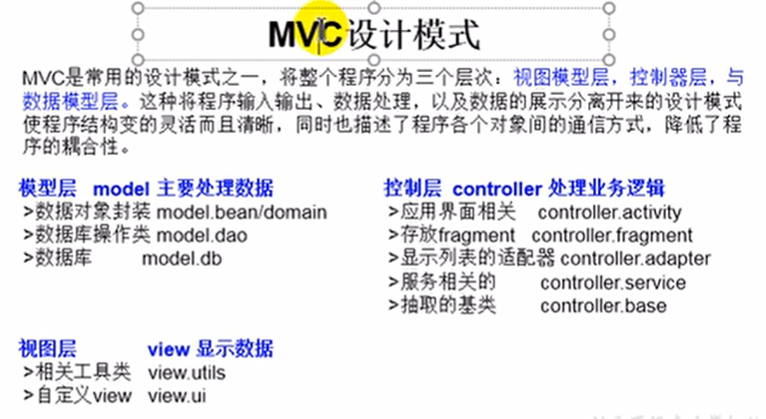### 热门文章## Java输出数组的内容## 母螳螂的“魅惑之术”## 疯狂填词 mad_libs 第9章9.9.2

#win7 python3.7.0 import os,reos.chdir(d:\documents\program_language) file1open(.\疯狂填词_d9z9d2_r.txt) file2open(.\疯狂填词_d9z9d2_w.txt,w) words[ADJECTIVE,NOUN,VERB,NOUN] str1file1.read()#方法1 for word in words :word_replaceinput(fEnter a {word} :)str1…## 分享你的看书记录

1、软件评测师教程&#xff08;纸质版&#xff09;共19章 2022.01.16 第1-7章、15章看完## HBASE 高可用## js事件操作语法## Photoshop插件--晕影动态--选区--脚本开发--PS插件## SQL练习问题汇总_及时更新## vs LNK1104 无法打开文件“xxx.obj”## 工业元宇宙的定义与实施路线图## 【leectode 2022.1.15】完成一半题目## js 面试题总结## java练习代码## RocketMQ-什么是死信队列？怎么解决## 项目 cg day04## 输出三角形

#include <stdio.h> int main() { int i,j; for(i0;i<5;i) { for(j0;j<i;j) { printf("*"); } printf("\n"); } }## 通过awk和shell来限制IP多次访问之学不会你打死我## Python - 如何像程序员一样思考